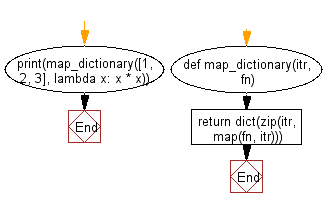﻿ Python: Map the values of a list to a dictionary using a function - w3resource# Python: Map the values of a list to a dictionary using a function

## Python List: Exercise - 220 with Solution

Write a Python program to map the values of a list to a dictionary using a function, where the key-value pairs consist of the original value as the key and the result of the function as the value.

• Use map() to apply fn to each value of the list.
• Use zip() to pair original values to the values produced by fn.
• Use dict() to return an appropriate dictionary.

Sample Solution:

Python Code:

``````def map_dictionary(itr, fn):
return dict(zip(itr, map(fn, itr)))
print(map_dictionary([1, 2, 3], lambda x: x * x))
```
```

Sample Output:

```{1: 1, 2: 4, 3: 9}
```

Flowchart:## Visualize Python code execution:

The following tool visualize what the computer is doing step-by-step as it executes the said program:

Python Code Editor:

Have another way to solve this solution? Contribute your code (and comments) through Disqus.

What is the difficulty level of this exercise?

Test your Python skills with w3resource's quiz

﻿

## Python: Tips of the Day

Floor Division:

When we speak of division we normally mean (/) float division operator, this will give a precise result in float format with decimals.

For a rounded integer result there is (//) floor division operator in Python. Floor division will only give integer results that are round numbers.

```print(1000 // 300)
print(1000 / 300)```

Output:

```3
3.3333333333333335```